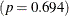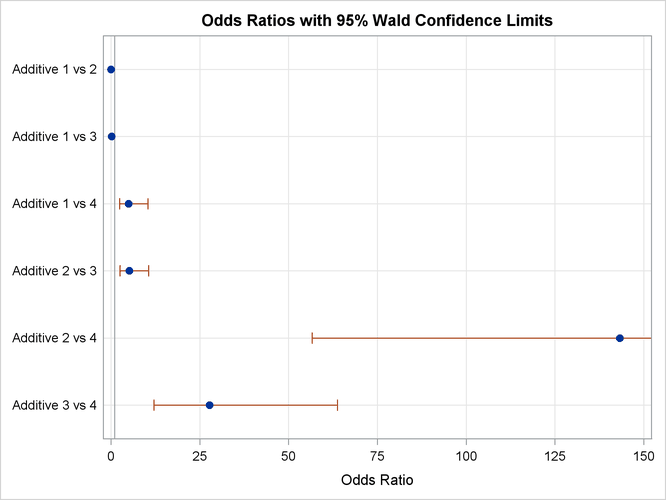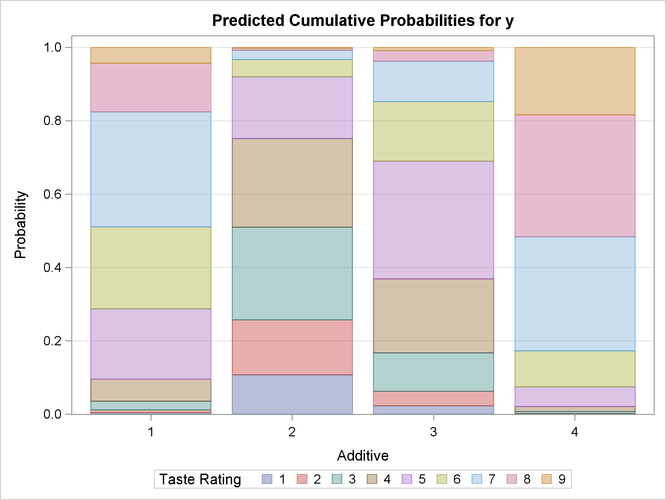### Example 54.3 Ordinal Logistic Regression

Consider a study of the effects on taste of various cheese additives. Researchers tested four cheese additives and obtained 52 response ratings for each additive. Each response was measured on a scale of nine categories ranging from strong dislike (1) to excellent taste (9). The data, given in McCullagh and Nelder (1989, p. 175) in the form of a two-way frequency table of additive by rating, are saved in the data set `Cheese` by using the following program. The variable `y` contains the response rating. The variable `Additive` specifies the cheese additive (1, 2, 3, or 4). The variable `freq` gives the frequency with which each additive received each rating.

```data Cheese;
do Additive = 1 to 4;
do y = 1 to 9;
input freq @@;
output;
end;
end;
label y='Taste Rating';
datalines;
0  0  1  7  8  8 19  8  1
6  9 12 11  7  6  1  0  0
1  1  6  8 23  7  5  1  0
0  0  0  1  3  7 14 16 11
;
```

The response variable `y` is ordinally scaled. A cumulative logit model is used to investigate the effects of the cheese additives on taste. The following statements invoke PROC LOGISTIC to fit this model with `y` as the response variable and three indicator variables as explanatory variables, with the fourth additive as the reference level. With this parameterization, each `Additive` parameter compares an additive to the fourth additive. The COVB option displays the estimated covariance matrix. The ODDSRATIO statement computes odds ratios for all combinations of the `Additive` levels. The PLOTS option produces a graphical display of the odds ratios, and the EFFECTPLOT statement displays the predicted probabilities.

```ods graphics on;
proc logistic data=Cheese plots(only)=oddsratio(range=clip);
freq freq;
effectplot / polybar;
title 'Multiple Response Cheese Tasting Experiment';
run;
ods graphics off;
```

The Response Profile table in Output 54.3.1 shows that the strong dislike (`y`=1) end of the rating scale is associated with lower Ordered Values in the Response Profile table; hence the probability of disliking the additives is modeled.

The score chi-square for testing the proportional odds assumption is 17.287, which is not significant with respect to a chi-square distribution with 21 degrees of freedom. This indicates that the proportional odds assumption is reasonable. The positive value (1.6128) for the parameter estimate for `Additive1` indicates a tendency toward the lower-numbered categories of the first cheese additive relative to the fourth. In other words, the fourth additive tastes better than the first additive. The second and third additives are both less favorable than the fourth additive. The relative magnitudes of these slope estimates imply the preference ordering: fourth, first, third, second.

Output 54.3.1: Proportional Odds Model Regression Analysis

 Multiple Response Cheese Tasting Experiment

The LOGISTIC Procedure

Model Information
Data Set WORK.CHEESE
Response Variable y Taste Rating
Number of Response Levels 9
Frequency Variable freq
Model cumulative logit
Optimization Technique Fisher's scoring

 Number of Observations Read 36 28 208 208

Response Profile
Ordered
Value
y Total
Frequency
1 1 7
2 2 10
3 3 19
4 4 27
5 5 41
6 6 28
7 7 39
8 8 25
9 9 12

Probabilities modeled are cumulated over the lower Ordered Values.

 Note: 8 observations having nonpositive frequencies or weights were excluded since they do not contribute to the analysis.

Class Level Information
Class Value Design Variables
2 0 1 0
3 0 0 1
4 0 0 0

Model Convergence Status
Convergence criterion (GCONV=1E-8) satisfied.

Score Test for the Proportional
Odds Assumption
Chi-Square DF Pr > ChiSq
17.2866 21 0.6936

Model Fit Statistics
Criterion Intercept Only Intercept and
Covariates
AIC 875.802 733.348
SC 902.502 770.061
-2 Log L 859.802 711.348

Testing Global Null Hypothesis: BETA=0
Test Chi-Square DF Pr > ChiSq
Likelihood Ratio 148.4539 3 <.0001
Score 111.2670 3 <.0001
Wald 115.1504 3 <.0001

Type 3 Analysis of Effects
Effect DF Wald
Chi-Square
Pr > ChiSq

Analysis of Maximum Likelihood Estimates
Parameter   DF Estimate Standard
Error
Wald
Chi-Square
Pr > ChiSq
Intercept 1 1 -7.0801 0.5624 158.4851 <.0001
Intercept 2 1 -6.0249 0.4755 160.5500 <.0001
Intercept 3 1 -4.9254 0.4272 132.9484 <.0001
Intercept 4 1 -3.8568 0.3902 97.7087 <.0001
Intercept 5 1 -2.5205 0.3431 53.9704 <.0001
Intercept 6 1 -1.5685 0.3086 25.8374 <.0001
Intercept 7 1 -0.0669 0.2658 0.0633 0.8013
Intercept 8 1 1.4930 0.3310 20.3439 <.0001
Additive 1 1 1.6128 0.3778 18.2265 <.0001
Additive 2 1 4.9645 0.4741 109.6427 <.0001
Additive 3 1 3.3227 0.4251 61.0931 <.0001

Association of Predicted Probabilities and
Observed Responses
Percent Concordant 67.6 Somers' D 0.578
Percent Discordant 9.8 Gamma 0.746
Percent Tied 22.6 Tau-a 0.500
Pairs 18635 c 0.789

The odds ratio results in Output 54.3.2 show the preferences more clearly. For example, the Additive 1 vs 4 odds ratio says that the first additive has 5.017 times the odds of receiving a lower score than the fourth additive; that is, the first additive is 5.017 times more likely than the fourth additive to receive a lower score. Output 54.3.3 displays the odds ratios graphically; the range of the confidence limits is truncated by the RANGE=CLIP option, so you can see that 1 is not contained in any of the intervals.

Output 54.3.2: Odds Ratios of All Pairs of Additive Levels

Odds Ratio Estimates and Wald Confidence Intervals
Label Estimate 95% Confidence Limits
Additive 1 vs 2 0.035 0.015 0.080
Additive 1 vs 3 0.181 0.087 0.376
Additive 1 vs 4 5.017 2.393 10.520
Additive 2 vs 3 5.165 2.482 10.746
Additive 2 vs 4 143.241 56.558 362.777
Additive 3 vs 4 27.734 12.055 63.805

Output 54.3.3: Plot of Odds Ratios for AdditiveThe estimated covariance matrix of the parameters is displayed in Output 54.3.4.

Output 54.3.4: Estimated Covariance Matrix

Estimated Covariance Matrix
Intercept_1 0.316291 0.219581 0.176278 0.147694 0.114024 0.091085 0.057814 0.041304 -0.09419 -0.18686 -0.13565
Intercept_2 0.219581 0.226095 0.177806 0.147933 0.11403 0.091081 0.057813 0.041304 -0.09421 -0.18161 -0.13569
Intercept_3 0.176278 0.177806 0.182473 0.148844 0.114092 0.091074 0.057807 0.0413 -0.09427 -0.1687 -0.1352
Intercept_4 0.147694 0.147933 0.148844 0.152235 0.114512 0.091109 0.05778 0.041277 -0.09428 -0.14717 -0.13118
Intercept_5 0.114024 0.11403 0.114092 0.114512 0.117713 0.091821 0.057721 0.041162 -0.09246 -0.11415 -0.11207
Intercept_6 0.091085 0.091081 0.091074 0.091109 0.091821 0.09522 0.058312 0.041324 -0.08521 -0.09113 -0.09122
Intercept_7 0.057814 0.057813 0.057807 0.05778 0.057721 0.058312 0.07064 0.04878 -0.06041 -0.05781 -0.05802
Intercept_8 0.041304 0.041304 0.0413 0.041277 0.041162 0.041324 0.04878 0.109562 -0.04436 -0.0413 -0.04143
Additive1 -0.09419 -0.09421 -0.09427 -0.09428 -0.09246 -0.08521 -0.06041 -0.04436 0.142715 0.094072 0.092128
Additive2 -0.18686 -0.18161 -0.1687 -0.14717 -0.11415 -0.09113 -0.05781 -0.0413 0.094072 0.22479 0.132877
Additive3 -0.13565 -0.13569 -0.1352 -0.13118 -0.11207 -0.09122 -0.05802 -0.04143 0.092128 0.132877 0.180709

Output 54.3.5 displays the probability of each taste rating `y` within each additive. You can see that `Additive`=1 mostly receives ratings of 5 to 7, `Additive`=2 mostly receives ratings of 2 to 5, `Additive`=3 mostly receives ratings of 4 to 6, and `Additive`=4 mostly receives ratings of 7 to 9, which also confirms the previously discussed preference orderings.

Output 54.3.5: Model-Predicted Probabilities